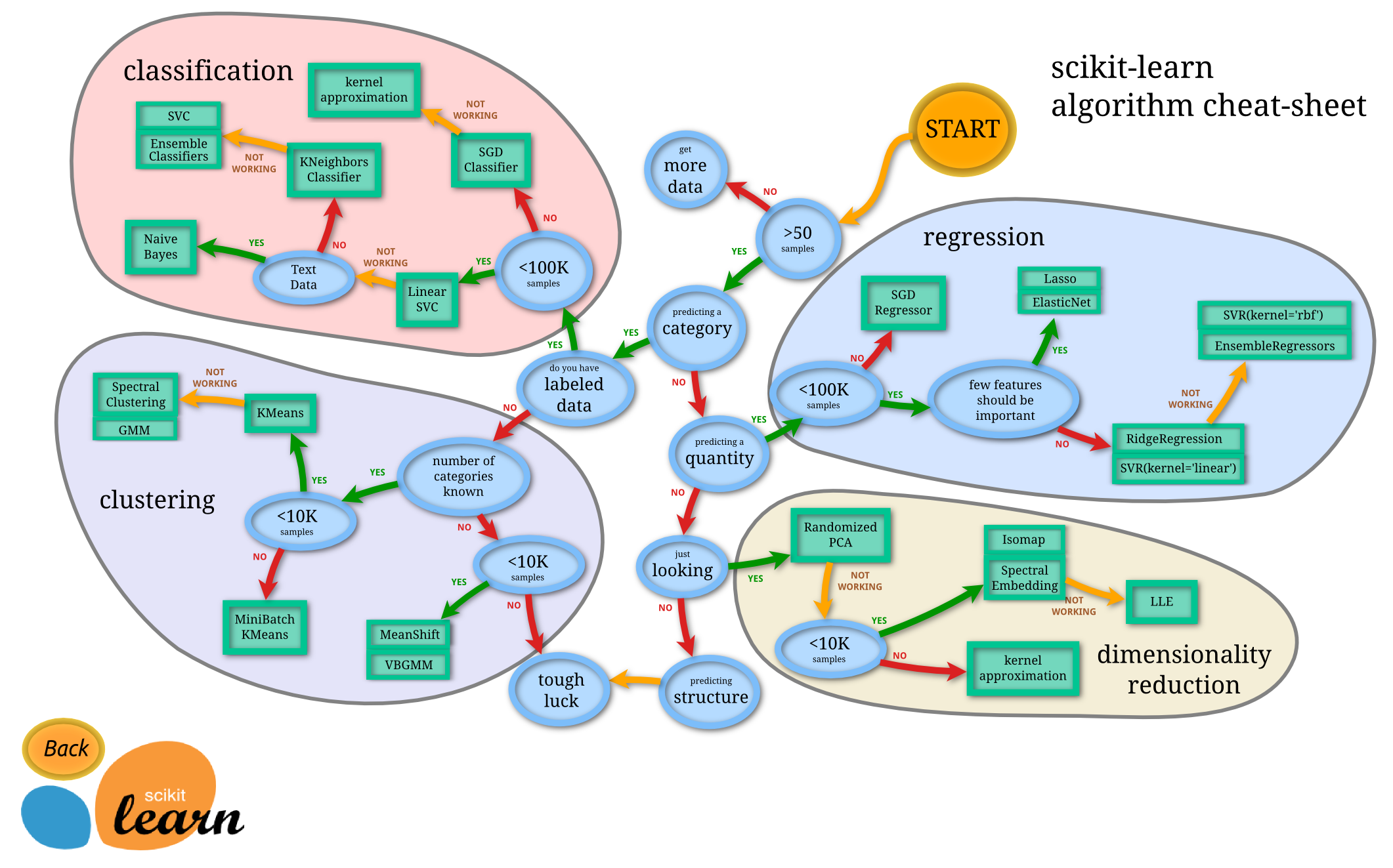# scikit-learn-intro¶

Credits: Forked from PyCon 2015 Scikit-learn Tutorial by Jake VanderPlas

• Machine Learning Models Cheat Sheet
• Estimators
• Introduction: Iris Dataset
• K-Nearest Neighbors Classifier
In :
%matplotlib inline
import numpy as np
import matplotlib.pyplot as plt
import seaborn;
from sklearn.linear_model import LinearRegression
from scipy import stats
import pylab as pl

seaborn.set()


## Machine Learning Models Cheat Sheet¶

In :
from IPython.display import Image
Image("http://scikit-learn.org/dev/_static/ml_map.png", width=800)

Out:## Estimators¶

Given a scikit-learn estimator object named model, the following methods are available:

• Available in all Estimators
• model.fit() : fit training data. For supervised learning applications, this accepts two arguments: the data X and the labels y (e.g. model.fit(X, y)). For unsupervised learning applications, this accepts only a single argument, the data X (e.g. model.fit(X)).
• Available in supervised estimators
• model.predict() : given a trained model, predict the label of a new set of data. This method accepts one argument, the new data X_new (e.g. model.predict(X_new)), and returns the learned label for each object in the array.
• model.predict_proba() : For classification problems, some estimators also provide this method, which returns the probability that a new observation has each categorical label. In this case, the label with the highest probability is returned by model.predict().
• model.score() : for classification or regression problems, most (all?) estimators implement a score method. Scores are between 0 and 1, with a larger score indicating a better fit.
• Available in unsupervised estimators
• model.predict() : predict labels in clustering algorithms.
• model.transform() : given an unsupervised model, transform new data into the new basis. This also accepts one argument X_new, and returns the new representation of the data based on the unsupervised model.
• model.fit_transform() : some estimators implement this method, which more efficiently performs a fit and a transform on the same input data.

## Introduction: Iris Dataset¶

In :
from sklearn.datasets import load_iris

n_samples, n_features = iris.data.shape
print(iris.keys())
print((n_samples, n_features))
print(iris.data.shape)
print(iris.target.shape)
print(iris.target_names)
print(iris.feature_names)

['target_names', 'data', 'target', 'DESCR', 'feature_names']
(150, 4)
(150, 4)
(150,)
['setosa' 'versicolor' 'virginica']
['sepal length (cm)', 'sepal width (cm)', 'petal length (cm)', 'petal width (cm)']

In :
import numpy as np
import matplotlib.pyplot as plt

# 'sepal width (cm)'
x_index = 1
# 'petal length (cm)'
y_index = 2

# this formatter will label the colorbar with the correct target names
formatter = plt.FuncFormatter(lambda i, *args: iris.target_names[int(i)])

plt.scatter(iris.data[:, x_index], iris.data[:, y_index],
c=iris.target, cmap=plt.cm.get_cmap('RdYlBu', 3))
plt.colorbar(ticks=[0, 1, 2], format=formatter)
plt.clim(-0.5, 2.5)
plt.xlabel(iris.feature_names[x_index])
plt.ylabel(iris.feature_names[y_index]);